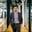Related Tags

# What are the characteristics of data structures?Muhammad Ahmad

Data structures are systematized ways of organizing data in order to use that data efficiently. There are some characteristics of every data structure that make it efficient. In this answer, we'll discuss those characteristics briefly and try to simulate them with basic examples. Let's have a look at the characteristics below.

### Characteristics of a data structure

There are three main characteristics:

• Correctness
• Time complexity
• Space complexity
Data structure's characteristics

### Correctness

This property is related to the algorithm of data structures. It's important that the algorithm is correct. Correctness here means that the algorithm always produces the expected output or follows the ground truth for the range of valid inputs, and eventually, it terminates. It is important because you're relying on it for the desired output.

### Types of correctness

There are further two types of correctness:

• Partial correctness
• Total correctness

#### Partial correctness

An algorithm is partially correct if it receives valid input and gets terminated. We can prove it through empirical analysisThe practice of using empirical methods to study the behavior of algorithms or by testing it on a few cases.

#### Total correctness

An algorithm is totally correct if it receives valid inputs and gets terminated, and always returns the correct output. We can prove this formal reasoning or mathematically, for instance, by proof by induction.

### Time complexity

The running time or execution time of a data structure as a function of the length of the input is known as time complexity.

It's a type of computation complexity that describe the time your algorithm needs to be executed. It should be as small as possible because the more time your data structure takes, it will decrease its efficiency.

Example: The access time complexity of an array is $O(1)$. This is because you can directly access any element of the array using its index.

### Space complexity

When an algorithm or data structure runs on your computer, it needs some sort of space/memory. The total memory space your data structure needs for its execution or to work properly is known as space complexity. Again, it should be as small as possible for data structures because it's one of the important factors in efficiency.

Example: A hashtable has a space complexity of$O(n)$.

Note: An efficient data structure execute quickly and save memory during the process.

RELATED TAGS

CONTRIBUTORMuhammad Ahmad
RELATED COURSES

View all Courses

Keep Exploring

Learn in-demand tech skills in half the time